Courses
Courses for Kids
Free study material
Free LIVE classes
More

# Who Is Heavier Class 3 Notes CBSE Maths Chapter 8 (Free PDF Download)LIVE
Join Vedantu’s FREE Mastercalss

## Easy Preparation of CBSE Class 3 Maths Chapter 8 Who is Heavier? Revision Notes and Worksheets

CBSE Class 3 Maths Chapter 8 Who is Heavier is an important part of this syllabus that students need to give prime attention to. This chapter explains how to measure weight and how to compare objects accordingly. To understand the context and concepts of this chapter well, download and refer to the revision notes developed by the subject experts of Vedantu. Use these notes to revise this chapter well before an exam to score more.

Class 3 Maths teaches students how to compare different objects according to their weights in Chapter 8. The pictorial representation of the stories introduces the concept of comparison of weights and asks students to solve certain questions. The use of Who is Heavier? revision notes will be the best bet to learn the principles and prepare this chapter well before an exam.

These revision notes have been compiled following the basic objective of this chapter. The students will find a simpler explanation of all the concepts. They can also prepare this chapter by solving the assigned worksheets in the revision notes.

Also, check CBSE Class 3 Maths revision notes for other chapters:

Last updated date: 24th Sep 2023
Total views: 144k
Views today: 1.44k

## Revision Notes Class 3 Maths Chapter 8 - Who’s Heavier?

### Weight

• Weight is the amount or quantity of heaviness or mass.

• The more the weight an object has heavier it is and vice versa.

• Example: 1 kg of oranges is less heavy than 3 kg of oranges.

### Measurement of Weight

• The weight of objects is normally measured in Kilograms.

• Simply, Kilograms can be represented as “kg”.

• Weights of Smaller objects are measured in Grams(g) and Milligrams(mg).

• 1 Milligram(mg) is less heavy than 1 Gram(g) and 1 Gram(g) is less heavy than 1 Kilogram(kg).

### Weight and Size

• If the weight of two quantities is the same, it is not always necessary that the size and shape of those two quantities are also the same.

• Example: Take 1 Kg Sugar and 1 Kg Popcorn. 1 Kg popcorn needs a larger bag when compared to 1 Kg Sugar bag.

• This is because Popcorn is lighter than sugar.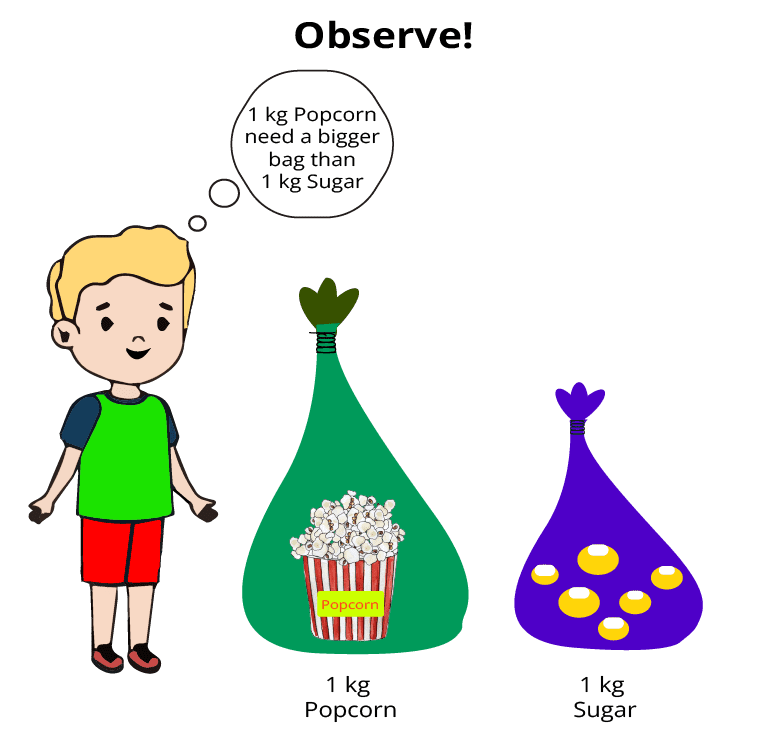Comparison of size of 1kg popcorn and 1kg sugar

• The size of objects does not always decide the weight.

• Example: The weight of an air-filled balloon is less than the weight of a small orange. (although their size appears the same)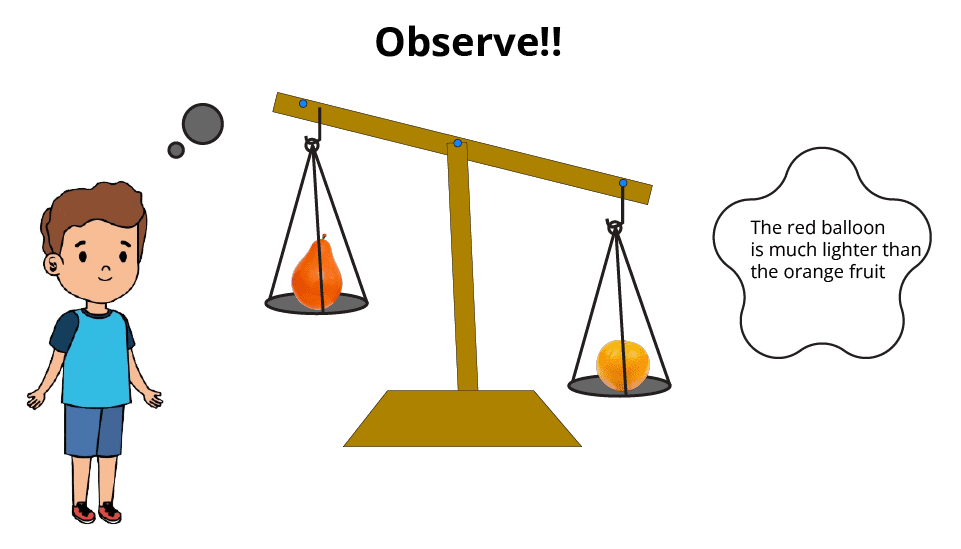Observation of weight comparison of a balloon and an orange

Solved Example 1: An elephant weighs 120 kg and a tiger weighs 100 kg. Which one is heavier and why?

Ans:  An elephant weighs more than a tiger therefore, the elephant is heavier than the tiger.

Solved Example 2: A watermelon weighs 6 kg and a mango weighs 1/2 kg. Which one is heavier and why? Also is it always true that an object which is bigger in size is heavier?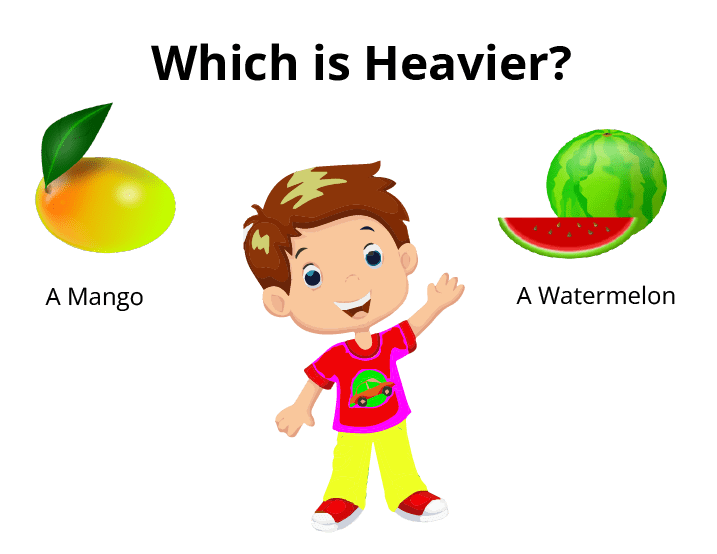A watermelon and a mango

Ans:  A watermelon weighs more than a mango therefore, the watermelon is heavier than the mango.

In this case, heavier fruit is bigger in size than smaller fruit.

No, it's not always true that a heavier object is bigger than a lighter object. Consider a packet of 1 kg of popcorn and 2 kg of an iron rod. The size of the popcorn packet will be more than the size of the iron rod but its weight will be less than the iron rod.

### Weights

• We use standard weights like 1kg, and 500 gm to measure the weight of other objects using balances.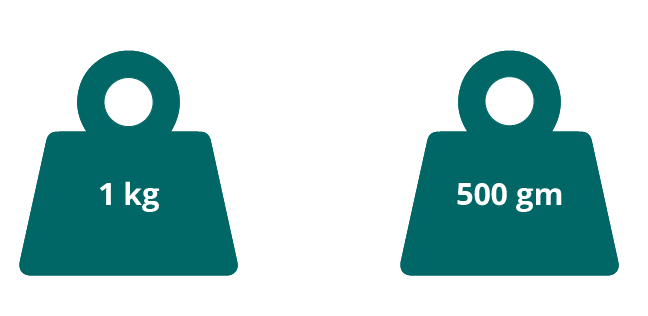Weights of 1kg and 500 gm

• Example: To measure the weight of 5 kg we can use five 1 kg weights or ten 500 grams weights to balance on the scale.

Solved Example 1: How many weights of 1 kg will be required to balance the weight of a 10 kg object?

Ans: We need ten 1 kg weights to balance the weight of the 10 kg object.

Scales

• We use scales to measure the weight of an object.

• There are different kinds of scales as shown in the below figure.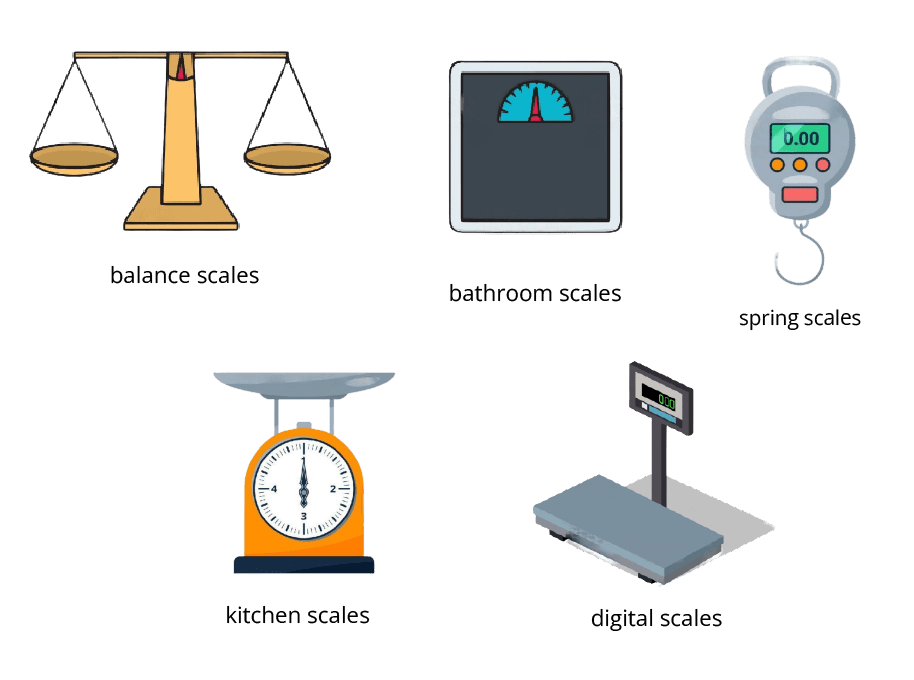Different scales to measure weight

•  Which kind of scale we should use depends on the amount of weight and the purpose of weighing.

• Example: A spring scale is used to measure the weight of a gas cylinder and bathroom scales are used to measure the weight of a person.

### Balance Scale

• Balance is a weighing device.

• It has a balanced beam as well as two pans.

• To determine the weight of an object, place it in one pan and standard weights in the other.

• An object which is heavier comes lower.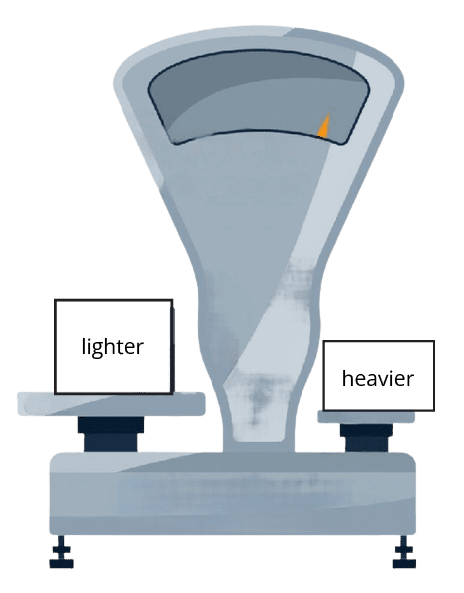A weight balance showing heavier and lighter sides

• The beam is in balance when the pans have the same weight.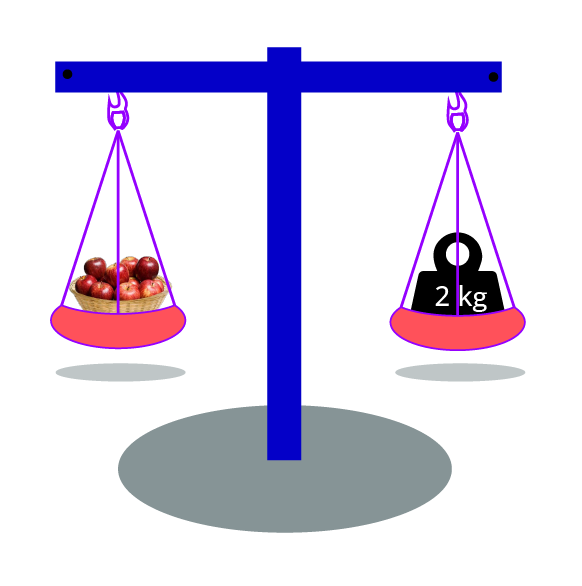A measure of equal weight on both sides of balance

• Example: In the above image weight of fruits is 2kg as the beam is balanced.

Solved Example 1: Using the below image, explain which fruit is heavier.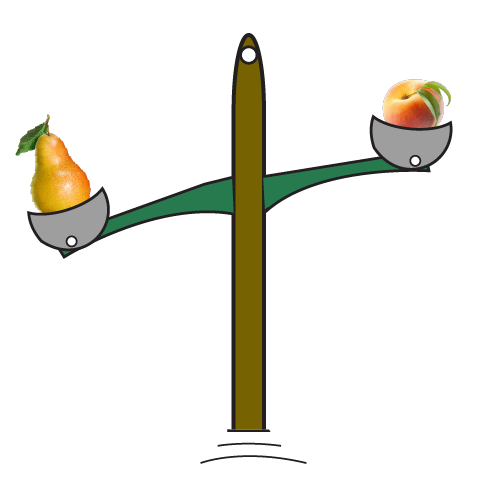Weight comparison of two fruits: pear and peach

Ans: Clearly Peach is heavier than Pear, as Peach is on the lower side.

### Practical applications of weights and balance

• When you buy fruits/vegetables from the market, the vendor uses balance to give the desired amount of fruits/vegetables.

• In order to weigh domestic gas cylinders, the use of a spring scale is very popular.

• Kitchen scales are used to weigh the number of particular components used in a recipe.

### Practice Question:

Q 1: The weight of a soft toy (made of cotton) is 1 kg whereas the weight of a car toy (made of steel) is 3 kg. Which one is heavier? Also, guess which is bigger in size.

Q 2: A steel chair weighs 15 kg and a wooden bed also weighs 15 kg. Comment on their sizes. (does equal weight implies their size will be the same).

Q  3: Which scale will you use to measure the weight of the gas cylinder?

Q 4: How many weights of 1 kg will be required to balance the weight of a 6 kg object?

Ans1. A steel car of 3 kg is heavier than a soft toy of 1 kg. There are high chances that soft toys will be bigger than cars as cotton is lighter than steel.

Ans2. Their size and shape can be different as they are made of different materials and for different use.

Ans3. A spring scale will be used to measure the weight of the gas cylinder.

Ans4. Six weights of 1 kg will be required.

## Why CBSE Class 3 Maths Chapter 8 Who is Heavier Importnat For Students?

This chapter, as mentioned earlier, introduces a new concept of weights. Students will learn to judge the weights of daily objects and compare them. The real-life examples are compiled in the form of real-life stories to make learning more fascinating. Here is why this chapter is important for the Class 4 students.

• Stories using gur and groundnuts help students realise the difference in weights of daily objects. It grows a sense of comparison between these objects. It leads the students to realise which is heavier than what.

• They will also learn to balance the weights on both sides of a see-saw using simple examples. They will use the concept of heavy and light weights for balancing activities.

• They will also take the first step of figuring out that objects with the same weight are actually equal to each other.

• The exercise questions also ask students to make guesses to check their imagination and logical reasoning power.

## Preparation Made Easier with Who is Heavier Revision Notes and Worksheets

Download the free worksheets and revision notes for Who is Heavier? and complete your study material. Make your exam preparation easier and better by revising the notes and solving worksheets.

## Importance of CBSE Class 3 Maths Chapter 8 Who is Heavier Revision Notes

The revision notes designed by the experts will ensure that students can grab hold of the concepts and mathematical principles related to the weights of objects well. By understanding these concepts and formulas, students will be able to use them to solve exercise problems without any hassle.

To make this process easier, the notes have been formulated in a simpler and more organized version. It will enable students to focus on the prime topics and revise the chapter faster. Hence, add these notes to the study material and take your preparation to the next level.

## Advantages of CBSE Class 3 Maths Chapter 8 Who is Heavier Revision Notes

• Get a consolidated file of revision notes here and access it whenever you want. You can also download the PDF version of these notes and make your study sessions more convenient.

• Resolve doubts related to the concepts of this chapter by using these notes and preparing them well.

• Follow the process of explaining the concepts and principles of weight in these notes to learn how to compile correct answers.

• Reduce the revision time before an exam by using these notes and then practice solving the exercise problems.

## Download CBSE Class 3 Maths Chapter 8 Who is Heavier Revision Notes PDF

Get the free PDF version of these notes and complete your study material. Refer to these notes according to your study and revision schedules. Use the simpler description of the concepts to understand the context of this chapter well. Utilise the organised format of these notes to recall what you have studied perfectly during an exam.

## FAQs on Who Is Heavier Class 3 Notes CBSE Maths Chapter 8 (Free PDF Download)

1. How can I solve questions related to pumpkin and tomato panga?

Find out what is heavier, the pumpkin or a tomato? It will help you determine how many lighter objects you need to balance the heavier object on a see-saw. This is how you can solve the questions.

2. Should I use NCERT solutions to prepare this chapter?

Thewill help you find the right answers. Learn how to write the answers from experts and then proceed to solve worksheets given with the revision notes.

3. How can I compare the weight of two objects given in a picture?

Identify the objects and use your common sense to differentiate and compare their weights. Read the question carefully and answer accordingly.

4. How can I understand the concepts of CBSE Class 3 Maths Chapter 8 Who is Heavier?

Focus on the notes designed by the experts to use the simpler version of all the concepts of this chapter. Learn to use them to solve exercise problems. Take help from the NCERT solutions for all the exercise questions and understand the concept well.

5. How can I use these notes before an exam?

Follow the content of this chapter first. Practise solving exercise questions again and refer to the notes to revise. You will be able to recall what you have studied and can compile accurate answers during an exam.